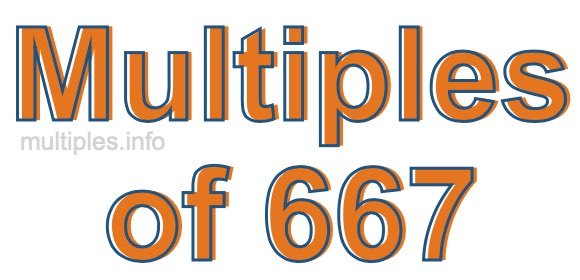Multiples of 667Welcome to the Multiples of 667 page. Here we will first teach you everything you will ever need to know about the multiples of 667, and then give you a study guide summary of everything we taught you to make sure you remember it all. Use this page to look up facts and learn information about the multiples of 667. This page will make you a multiples of six hundred sixty-seven expert!

Definition of Multiples of 667
Multiples of 667 are all the numbers that when divided by 667 equal an integer. Each of the multiples of 667 are called a multiple. A multiple of 667 is created by multiplying 667 by an integer.

Therefore, to create a list of multiples of 667, you start with 1 multiplied by 667, then 2 multiplied by 667, then 3 multiplied by 667, and so on for as long as you want. Thus, the list of the first five multiples of 667 is 667, 1334, 2001, 2668, and 3335. To see a larger list of multiples of 667, see the printable image of Multiples of 667 further down on this page. We also have a category where you can choose any nth multiple of 667.

Multiples of 667 Checker
The Multiples of 667 Checker below checks to see if any number of your choice is a multiple of 667. In other words, it checks to see if there is any number (integer) that when multiplied by 667 will equal your number. To do that, we divide your number by 667. If the the quotient is an integer, then your number is a multiple of 667.

Is  a multiple of 667?

Least Common Multiple of 667 and ...
A Least Common Multiple (LCM) is the lowest multiple that two or more numbers have in common. This is also called the smallest common multiple or lowest common multiple and is useful to know when you are adding our subtracting fractions. Enter one or more numbers below (667 is already entered) to find the LCM.

Check out our LCM Calculator if you need more details about the Least Common Multiple or if you need the LCM for different numbers for adding and subtraction fractions.

nth Multiple of 667
As we stated above, 667 is the first multiple of 667, 1334 is the second multiple of 667, 2001 is the third multiple of 667, and so on. Enter a number below to find the nth multiple of 667.

th multiple of 667

Multiples of 667 vs Factors of 667
667 is a multiple of 667 and a factor of 667, but that is where the similarities end. All postive multiples of 667 are 667 or greater than 667. All positive factors of 667 are 667 or less than 667.

Below is the beginning list of multiples of 667 and the factors of 667 so you can compare:

Multiples of 667: 667, 1334, 2001, 2668, 3335, etc.

Factors of 667: 1, 23, 29, 667

As you can see, the multiples of 667 are all the numbers that you can divide by 667 to get a whole number. The factors of 667, on the other hand, are all the whole numbers that you can multiply by another whole number to get 667.

It's also interesting to note that if a number (x) is a factor of 667, then 667 will also be a multiple of that number (x).

Multiples of 667 vs Divisors of 667
The divisors of 667 are all the integers that 667 can be divided by evenly. Below is a list of the divisors of 667.

Divisors of 667: 1, 23, 29, 667

The interesting thing to note here is that if you take any multiple of 667 and divide it by a divisor of 667, you will see that the quotient is an integer.

Multiples of 667 Table
Below is an image of the first 100 multiples of 667 in a table. The table is in chronological order, column by column. The first column has the first ten multiples of 667, the second column has the next ten multiples of 667, and so on.The Multiples of 667 Table is also referred to as the 667 Times Table or Times Table of 667. You are welcome to print out our table for your studies.

Negative Multiples of 667
Although not often discussed or needed in math, it is worth mentioning that you can make a list of negative multiples of 667 by multiplying 667 by -1, then by -2, then by -3, and so on, to get the following list of negative multiples of 667:

-667, -1334, -2001, -2668, -3335, etc.

Multiples of 667 Summary
Below is a summary of important Multiples of 667 facts that we have discussed on this page. To retain the knowledge on this page, we recommend that you read through the summary and explain to yourself or a study partner why they hold true.

There are an infinite number of multiples of 667.

A multiple of 667 divided by 667 will equal a whole number.

667 divided by a factor of 667 equals a divisor of 667.

The nth multiple of 667 is n times 667.

The largest factor of 667 is equal to the first positive multiple of 667.

667 is a multiple of every factor of 667.

667 is a multiple of 667.

A multiple of 667 divided by a divisor of 667 equals an integer.

667 divided by a divisor of 667 equals a factor of 667.

Any integer times 667 will equal a multiple of 667.

Multiples of a Number
Here you can get the multiples of another number, all with the same attention to detail as we did for multiples of 667 on this page.

Multiples of
Multiples of 668
Did you find our page about multiples of six hundred sixty-seven educational? Do you want more knowledge? Check out the multiples of the next number on our list!